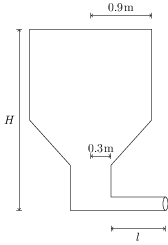# Viscosity

## Problems from IIT JEE

Problem (IIT JEE 2003):A liquid of density ${900}\;\mathrm{kg/m^3}$ is filled in a cylindrical tank of upper radius $0.9\;\mathrm{m}$ and lower radius ${0.3}\;\mathrm{m}$. A capillary tube of length $l$ is attached at the bottom of the tank as shown in the figure. The capillary has outer radius ${0.002}\;\mathrm{m}$ and inner radius $a$. When pressure $p$ is applied at the top of the tank volume flow rate of the liquid is ${8\times{10}^{-6}}\;{m^3/s}$ and if capillary tube is detached, the liquid comes out from the tank with a velocity of ${10}\;\mathrm{m/s}$. Determine the coefficient of viscosity of the liquid. (Given : $\pi a^2={{10}^{-6}}\;\mathrm{m^2}$ and $a^2/l={2\times{10}^{-6}}\;\mathrm{m}$.)

Solution: Let $r_1={0.9}\mathrm{m}$ be the radius at the top, $v_1$ be the velocity at the top, $r_2=0.3\;\mathrm{m}$ be the radius at the bottom, and $v_2={10}\;{m/s}$ be the velocity at the bottom (when capillary tube is detached). The continuity equation, $\pi r_1^2 v_1=\pi r_2^2 v_2$, gives $v_1={{10}/{9}}\;{m/s}$. Let $p_0$ be the atmospheric pressure. Apply Bernoulli's equation between the top and bottom, \begin{align} p_0+p+\rho g H+\tfrac{1}{2}\rho v_2^2=p_0+0+\tfrac{1}{2}\rho v_1^2, \end{align} to get, \begin{align} p+\rho g H=\tfrac{1}{2}\rho(v_2^2-v_1^2)={\tfrac{4}{9}\times{10}^{5}}\mathrm{N/m^2}. \end{align} The pressure difference across the capillary is, \begin{align} \Delta p=(p_0+p+\rho gH)-p_0=p+\rho g H. \end{align} By Poiseuille's equation, volume flow rate in a capillary is, \begin{align} \label{glb:eqn:4} Q=\frac{\pi \Delta p a^4}{8\eta l}=\frac{\Delta p (\pi a^2) (a^2/l)}{8\eta}. \end{align} Substitute the values in above equation to get $\eta={\frac{1}{720}}\;{N\,s/m^2}$.